Example Questions

Example Question #31 : Data Analysis

If you throw a die three times, what is the probability of getting a 1 on at least one of the three throws?

5/6

25/36

1/3

125/216

91/216

91/216

Explanation:

Let's first find the probability of not getting a 1 on any of the three throws. Prob(no 1 on first throw) = 5/6. We need Prob(no 1 on first throw AND no 1 on second throw AND no 1 on third throw). "And" signifies multiplication in probability, so Prob(no 1 on all three throws) = 5/6 * 5/6 * 5/6 = 125/216. Now, to find the probability of getting a 1 on at least one throw, we simply take 1 – Prob(no 1 on all three throws) = 1 – 125/216 = 91/216.

Example Question #21 : Outcomes

Every day is either rainy or sunny. Mondays are rainy with probability 3/5. Tuesdays are sunny with probability 1/4. Wednesdays are also sunny with probability 2/3. What is the probability that the weather is the same on Monday, Tuesday, and Wednesday?

1/8

13/60

1/15

15/19

3/20

13/60

Explanation:

We want either rainy, rainy, rainy or sunny, sunny, sunny.

P(rain on Mon AND rain on Tues AND rain on Wed) = 3/5 * 3/4 * 1/3 = 9/60

P(sun on Mon AND sun on Tues AND sun on Wed) = 2/5 * 1/4 * 2/3 = 4/60

P(same weather) = P(all 3 rainy days OR all 3 sunny days)

= P(all 3 rainy days) + P(all 3 sunny days)

= 9/60 + 4/60 = 13/60.

Example Question #21 : Probability

16 dogs and 21 cats are up for adoption at the pound. 12 of these animals are brown and 19 are female. What is the probability of randomly selecting a brown animal?

19/37

Cannot be determined

12/37

16/19

12/19

12/37

Explanation:

The problem gives us extra information. We know 12 animals are brown, and there are 16 + 21 = 37 animals in total. Then the probability of choosing a brown animal is simply 12/37.

Example Question #22 : Probability

What is the probability of pulling 2 spades out of a standard deck of cards without replacement?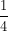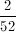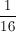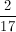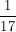Explanation:

There are 52 cards in a standard deck. 13 of them are spades.

So there is a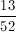chance of pulling out the first spade. That leaves 51 cards and 12 spades left. Now there is a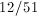chance to get a second spade.

When you combine probabilities, multiply the two individual probabilites together.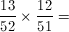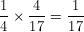Example Question #21 : Probability

What is the probability of fliiping a coin 4 times and getting 2 heads.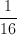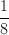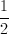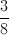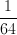Explanation:

There are 4 flips of the coin.chance that the first is a heads, the second is a heads, the third is a tails, and the fourth is a tails. There are 6 permutations that give a combined 2 heads and 2 tails in whatever order.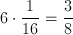Example Question #21 : Probability

A bag contains 4 blue marbles, 2 black marbles, 1 clear marble, and 3 red marbles. What is the probability of pulling out 3 red marbles without replacement?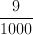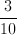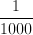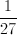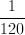Explanation:

There are 10 marbles in the bag. So, the first probability of pulling out the first red marble is; the next is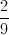; the final is.

Multiply the individual probabilities together to find the total outcome.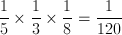Example Question #31 : Data Analysis

A study was conducted to determine the effectiveness of a vaccine for the common cold (Rhinovirus sp.). 1000 patients were studied. Of those, 500 received the vaccine and 500 did not. The patients were then exposed to the Rhinovirus and the results were tabulated.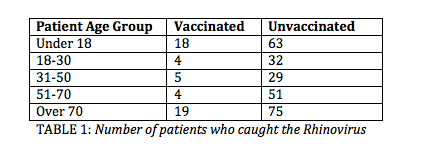Table 1 shows the number of vaccinated and unvaccinated patients in each age group who caught the cold.

In order to determine the effectiveness of the vaccine, scientists want to show the impact of the vaccine on a patient's odds of catching a cold.

A patient who receives the vaccination is __________ to catch Rhinovirus than an unvaccinated patient.

80% more likely

just as likely

20% more likely

20% less likely

80% less likely

80% less likely

Explanation:

According to the table, 50 unvaccinated patients caught Rhinovirus.

18 + 4 + 5 + 4 + 19 = 50

250 unvaccinated patients caught Rhinovirus.

63 + 32 + 29 + 51 + 75 = 250

Next, we need to determine the percentage relationship between the number of unvaccinated versus vaccinated patients that caught the virus.

50/250 = 20%

100% – 20% = 80%

Because only 20% as many vaccinated patients exposed to the cold virus caught the cold, this reflects an 80% reduction in the likelihood of a patient to catch the cold after receiving the vaccine.

Example Question #22 : Probability

A standard deck of cards contains 52 cards and 13 of each suit. Megan chooses two cards, without replacing. What is the probability that Megan chooses two diamonds?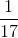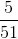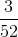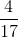Explanation:

For the first card, the probability of choosing a diamond is. With no replacing, the probability of choosing another diamond is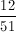. Multilying these probabilities yields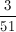.

This reduces to.

Example Question #31 : Probability

You see someone correctly guess a card from a 52 card deck and you want to out-do him in an equally "odds-defying" feat. How many sequential coin flips would you have to predict to do something "less-likely" than guessing the correct card from a 52 card deck?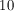Explanation:

You are trying to have "worse" odds than a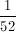chance. 5 correct coin flip guesses would be a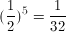chance, not quite as unlikely.

6 correct guesses however would be a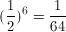chance, thus "outdoing" the odds of the card guess.

Example Question #33 : Data Analysis

A fair six-sided die is thrown.

Quantity A: The probability that the die will show either a "6" or an odd number.

Quantity B: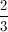Quantity B is greater.

Quantity A is greater.

The relationship cannot be determined from the information given.

The two quantities are equal.

To obtain probability, you must divide the number of possible desired outcomes by the number of total possible outcomes. The number of desired outcomes is 4 (3 odd numbers plus 1 even number). The number of total outcomes is 6 (number of sides on the die). So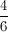reduces to, making the 2 quantities equal.